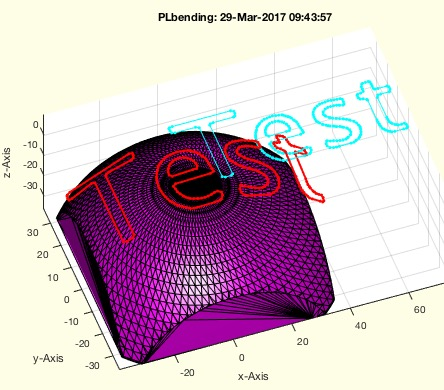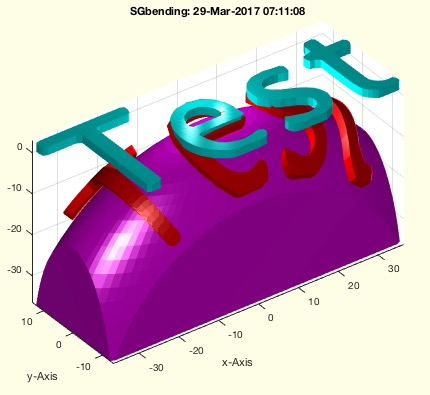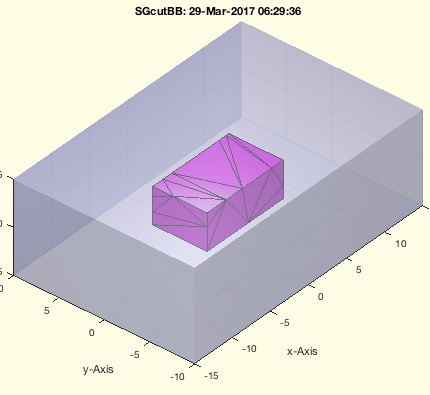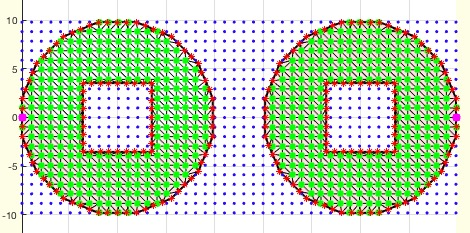% PLbending(PL,Rx,de,Ry,Rz,cent) - bends a CPL on a cylinder or spherical ellipsoid% (by Tim Lueth, VLFL-Lib, 2017-MÄR-29 as class: SURFACES)% % This fnctn bends closed polygon line on a radial sphere (cylinder or % ball). The original CPL is not modified, i.e. it is not intended to add % additional points on the surface before the bending: % Use CPLaddauxpoints to add points on the contour.% Use PLFLofCPLdelaunayGrid to add points on the surface% (Status of: 2017-03-29)% % See also: CPLaddauxpoints, PLFLofCPLdelaunayGrid, SGbending% % PLN=PLbending(PL,[Rx,de,Ry,Rz,cent])% === INPUT PARAMETERS ===% PL: Point List% Rx: Radius Rx% de: distance/intrusion; default is -0.1% Ry: Optional radius Ry; default is 10000% Rz: Optional radius Rz; default is Rx% cent: center solid before bending; default is true% === OUTPUT RESULTS ======% PLN: Vertex list of the bended point list% % EXAMPLE: Bends a CPL 1 mm above a sphere% CPL=CPLoftext('Test'); PLbending(CPLaddauxpoints(CPL,1),50,10,50,50);% [PL,FL,EL]=PLFLofCPLdelaunayGrid(CPL,1,1,1); VLFLplot(PL,FL);% PL=PLbending(PL,50,10,50,50); VLFLplot(PL,FL);%% SGbending(SG,Rx,de,Ry,Rz,cent) - beates a solid geoemtry on a cylinder or spherical ellipsoid% (by Tim Lueth, VLFL-Lib, 2017-MÄR-29 as class: SURFACES)% % This fnctn bends a solid geometry on a radial sphere (cylinder or % ball). The original solid is not modified, i.e. it is not intended to % add additional points on the surface before the bending: % Use CPLaddauxpoints to add points on the contour % Use PLFLofCPLdelaunayGrid to add point on the surface% Use SGofCPLzdelaunayGrid to create solids with a grid% (Status of: 2017-03-29)% % See also: SGofCPLzdelaunayGrid, SGofCPLsphere, CPLaddauxpoints, % PLFLofCPLdelaunayGrid% % SGN=SGbending(SG,[Rx,de,Ry,Rz,cent])% === INPUT PARAMETERS ===% SG: Solid Geometry% Rx: Radius Rx% de: distance/intrusion; default is -0.1% Ry: Optional radius Ry; default is 10000% Rz: Optional radius Rz; default is Rx% cent: center solid before bending; default is true% === OUTPUT RESULTS ======% SGN: Solid Geometry% % EXAMPLE: Bends a surface 1 mm above a sphere% SG=SGofCPLzdelaunayGrid(CPLoftext('Test'),2,1,1,1);% SGbending(SG,50,1,20,50)% SGbending(SG,50,1,20,50,false)%% SGcutBB(SG,ax,de) - cuts out a bounding box from a solid% (by Tim Lueth, VLFL-Lib, 2017-MÄR-29 as class: SURFACES)% % THis fnctn uses SGcut for creating the output result, not SGbool. It % must be clear, that this fnctn creates a lot of additional points at % the surfaces (Status of: 2017-03-29)% % See also: SGcut, SGbool% % SGN=SGcutBB(SG,ax,[de])% === INPUT PARAMETERS ===% SG: Solid geometry% ax: axis, such as Bounding box% de: delta to add on each side; default is 0;% === OUTPUT RESULTS ======% SGN: resulting solid% % EXAMPLE: % SGcutBB (SGbox([30,20,10]),[0 5 -2 2 0 4])% SGcutBB (SGbox([30,20,10]),[-inf inf -3 3 -2 2])% A=SGbool('x',SGbox([30,20,10]),SGofBB([-1000 1000 -3 3 -2 2])); % SGfigure(A)%% insidePLELdelaunay(PL,EL,GPL) - returns whether a point is inside/outside a contour% (by Tim Lueth, VLFL-Lib, 2017-MÄR-28 as class: ANALYTICAL GEOMETRY)% % This fnctn use delaunayTriangulation and makes sense only for larger % grid point list not for individual Points. Works only with 2D point % list and a correct contour edge list. (Status of: 2017-03-28)% % See also: insidePLEL% % [ki,XVL,XFL,XEL]=insidePLELdelaunay(PL,EL,GPL)% === INPUT PARAMETERS ===% PL: 2D Point list% EL: Contour edge list% GPL: Grid point list or Point list% === OUTPUT RESULTS ======% ki: +1==inside, -1==outside, 0== on the contour% XVL: Point List% XFL: Face List% XEL: Edge list% % EXAMPLE: % CPL=CPLaddauxpoints(CPLsample(12),1);% [PL,EL]=PLELofCPL(CPL);% GPL = GPLauxgridpointsPLEL(PL,EL,1,1);% insidePLELdelaunay(PL,EL,GPL);%